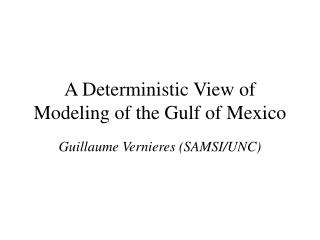DownloadDownload PresentationA Deterministic View of Modeling of the Gulf of Mexico

# A Deterministic View of Modeling of the Gulf of Mexico

Download Presentation## A Deterministic View of Modeling of the Gulf of Mexico

- - - - - - - - - - - - - - - - - - - - - - - - - - - E N D - - - - - - - - - - - - - - - - - - - - - - - - - - -
##### Presentation Transcript

1. A Deterministic View of Modeling of the Gulf of Mexico Guillaume Vernieres (SAMSI/UNC)

2. Outline • Motivations • Some physical background • Mathematical formulation of the problem • Results • …That’s it …

3. Motivations • Why do we care? http://www.camex4.com/photos/Ivan.A2004258.1635.2km.jpg

4. Motivations • Why do we care? HURRICANE TRACK PREDICTION !!!!!!!!!

5. Motivations • Why do we care? Test bed for modeling methods

6. Physical background • Ocean currents http://www.waterencyclopedia.com/images/wsci_03_img0381.jpg

7. Physical background • Global Wind http://research.utep.edu/Portals/72/weather%20NOAA/global%20wind.gif

8. Physical background • The Gulf Stream

9. Physical background • The Gulf of Mexico: Shedding of eddies Sea Surface Height in cm

10. Physical background • The Gulf of Mexico: Shedding of eddies Sea Surface Temperature

11. Mathematical formulation of the problem

12. Mathematical formulation of the problem

13. Mathematical formulation of the problem

14. Mathematical formulation of the problem Simple conservation laws:

15. Mathematical formulation of the problem Simple conservation laws: • Conservation of mass

16. Mathematical formulation of the problem Simple conservation laws: • Conservation of mass =

17. Mathematical formulation of the problem Simple conservation laws: • Conservation of mass • Conservation of momentum

18. Mathematical formulation of the problem Simple conservation laws: • Conservation of mass • Conservation of momentum • Rotating frame!! (yes the earth is turning!)

19. Mathematical formulation of the problem Simple conservation laws: • Conservation of mass • Conservation of momentum • Rotating frame!! (yes the earth is turning!) • Hydrostatic pressure

20. Mathematical formulation of the problem Simple conservation laws: • Conservation of mass • Conservation of momentum • Rotating frame!! (yes the earth is turning!) • Hydrostatic pressure • Neglect thermodynamics

21. Mathematical formulation of the problem Simple conservation laws: • Conservation of mass • Conservation of momentum • Rotating frame!! (yes the earth is turning!) • Hydrostatic pressure • Neglect thermodynamics • L>>D

22. Mathematical formulation of the problem Simple conservation laws: • Conservation of mass • Conservation of momentum • Rotating frame!! (yes the earth is turning!) • Hydrostatic pressure • Neglect thermodynamics • L>>D Similar to the Navier-Sokes equations

23. Mathematical formulation of the problem x & y momentum

24. Mathematical formulation of the problem Hydrostatic assumption

25. Mathematical formulation of the problem Continuity equation (conservation of mass)

26. Mathematical formulation of the problem

27. Mathematical formulation of the problem Can be further simplified !!

28. Mathematical formulation of the problem z u1=u1(x,y,t) ρ1=cst u2=u2(x,y,t) ρ2=cst>ρ1 ∞

29. Mathematical formulation of the problem Shallow water equations

30. Discretized in space using FiniteDifference

31. η x(ζ, η)=? y(ζ, η)=? ζ

32. Discretized in space using FiniteDifference • Discretized in time using Adams-Bashforth • 2nd order

33. 22500 grid points x 3 layers x 3 state variables (u,v,h)/layer = 202500 ODE’s

34. Some Results:

35. Eulerian and Lagrangian results

36. How much information is contained in one single drifter ?

37. Higher Re

38. “Influence of a drifter on the state of the GoM”

39. “Influence of a drifter on the state of the GoM”